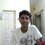# A strange behavior of numbers

Let there be m real numbers greater than 1, be called a set S. If we reciprocate every element in set S, all the numbers will lie between 0 to 1. Hence, number of real numbers between 0 to 1 is m.

1.Now, let us consider the number of real number between 1 to 2 be n. It is obvious that m>n.

2.If we add 1 to all the elements in set S we get a new set of real number (be called a set Q) which has all it's elements between 1 to 2 and the number of elements is m (as we are adding 1 to all the elements in set S).

In para (1) we are saying number of that the number of real numbers between 1 to 2 is n (which is less than m) and in para (2) we are saying that number of real number between 1 to 2 is m.

Why are these two contradicting each other?

And how can there be equal number of real number between 0 to 1 and 1 to infinity?Note by Rahul Dandwate
6 years, 11 months ago

This discussion board is a place to discuss our Daily Challenges and the math and science related to those challenges. Explanations are more than just a solution — they should explain the steps and thinking strategies that you used to obtain the solution. Comments should further the discussion of math and science.

When posting on Brilliant:

• Use the emojis to react to an explanation, whether you're congratulating a job well done , or just really confused .
• Ask specific questions about the challenge or the steps in somebody's explanation. Well-posed questions can add a lot to the discussion, but posting "I don't understand!" doesn't help anyone.
• Try to contribute something new to the discussion, whether it is an extension, generalization or other idea related to the challenge.

MarkdownAppears as
*italics* or _italics_ italics
**bold** or __bold__ bold
- bulleted- list
• bulleted
• list
1. numbered2. list
1. numbered
2. list
Note: you must add a full line of space before and after lists for them to show up correctly
paragraph 1paragraph 2

paragraph 1

paragraph 2

[example link](https://brilliant.org)example link
> This is a quote
This is a quote
    # I indented these lines
# 4 spaces, and now they show
# up as a code block.

print "hello world"
# I indented these lines
# 4 spaces, and now they show
# up as a code block.

print "hello world"
MathAppears as
Remember to wrap math in $$ ... $$ or $ ... $ to ensure proper formatting.
2 \times 3 $2 \times 3$
2^{34} $2^{34}$
a_{i-1} $a_{i-1}$
\frac{2}{3} $\frac{2}{3}$
\sqrt{2} $\sqrt{2}$
\sum_{i=1}^3 $\sum_{i=1}^3$
\sin \theta $\sin \theta$
\boxed{123} $\boxed{123}$

Sort by:

This is opening a really big topic, and one which raises some very difficult ideas. We say that two sets have the same number of elements if we can find a one-to-one correspondence between their elements. A one-to-one correspondence between sets $A$ and $B$ is, basically, a function from $A$ to $B$ such that

• distinct elements of $A$ are mapped to distinct elements of $B$,

• every element of $B$ is the image of some element of $A$.

This reasonable suggestion has apparently weird consequences. For example, there are as many positive integers as there are even positive integers, since the correspondence $n \mapsto 2n$ puts the positive integers and the even positive integers into one-to-one correspondence. It "gets worse", since there are as many rational numbers as there are integers!.

It turns out that there are more real numbers than there are integers. However, there are the same number of real numbers between $0$ and $1$ as there are between $0$ and $2$, with the map $x \mapsto 2x$ being the one-to-one correspondence we need.

The function $f(x) = \tfrac{x}{1+x}$ provides a one-to-one correspondence between the positive real numbers and the positive real numbers less than $1$, so these two sets have the same number of elements.

If you want to play with some of the ideas relating to infinity, try searching for information on Hilbert's Hotel on the web.

- 6 years, 11 months ago

"It is obvious that m > n." Is it?

- 6 years, 11 months ago

It should be as a whole is always greater than part of it.

- 6 years, 11 months ago

That's the part where your argument breaks down. With infinities, a whole is not necessarily greater than the part of it, although it's never less. In this particular case, turns out the whole is the same as the part.

- 6 years, 11 months ago

Your argument fails at the first condition itself. $m$ is not necessarily greater than $n$; a one-to-one correspondence could be established.

Sometimes it is not so easy to imagine this by plainly looking at sets; a picture is sometimes necessary. You'll be interested by the bottom portion of this diagram. Imagine each point on the semicircle representing a real value between 0 and 1 (with 0 and 1 represented by the endpoints of the diameter of the semicircle). Each point on the line represents a real number (with the left side going towards negative infinity and the right side going towards positive infinity). If one draws any line from the center of the semicircle to the line below it, exactly two points or no points are encountered for every possible line drawn. Thus, a one-to-one correspondence can be established between the real numbers in any finite range and the real numbers in an unbounded range.

I leave the exercise to you to try to find the one-to-one correspondence between the sets described above (I highly suggest a geometrical approach.)

- 6 years, 11 months ago